An air bubble in a sphere having 4 cm diameter that appears 1 cm from the surface nearest to the eye when looked along diameter. If${}_{a}\mu _{g}$ = 1.5, the distance of bubble from the refracting surface is

(a) 1.2 cm                    (b) 3.2 cm

(c) 2.8 cm                    (d) 1.6 cm

Concept Questions :-

Lenses
High Yielding Test Series + Question Bank - NEET 2020

Difficulty Level:

An observer can see through a pinhole the top end of a thin rod of height h, placed as shown in the figure. The beaker height is 3h and its radius h. When the beaker is filled with a liquid up to a height 2h, he can see the lower end of the rod. Then the refractive index of the liquid is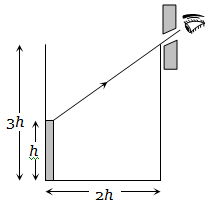(a) $5/2$                                    (b) $\sqrt{\left(5/2\right)}$

(c) $\sqrt{\left(3/2\right)}$                               (d) 3/2

Concept Questions :-

Refraction at plane surface
High Yielding Test Series + Question Bank - NEET 2020

Difficulty Level:

In an experiment of find the focal length of a concave mirror a graph is drawn between the magnitudes of u and v.  The graph looks like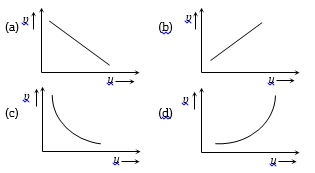Concept Questions :-

Reflection at spherical surface
High Yielding Test Series + Question Bank - NEET 2020

Difficulty Level:

As the position of an object (u) reflected from a concave mirror is varied, the position of the image (v) also varies. By letting the u change from 0 to infinity, the graph between v versus u will be-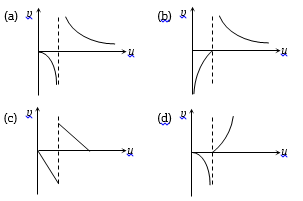Concept Questions :-

Reflection at spherical surface
High Yielding Test Series + Question Bank - NEET 2020

Difficulty Level:

The graph between u and v for a convex mirror is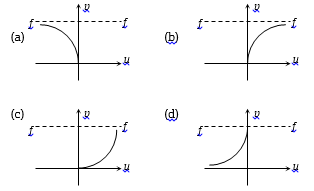Concept Questions :-

Reflection at spherical surface
High Yielding Test Series + Question Bank - NEET 2020

Difficulty Level:

A glass prism $\left(\mu =1.5\right)$ is dipped in water $\left(\mu =4/3\right)$ as shown in figure. A light ray is incident normally on the surface AB. It reaches the surface BC after totally reflected, if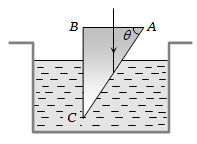(a)                             (b)

(c)                             (d) It is not possible

Concept Questions :-

Total internal reflection
High Yielding Test Series + Question Bank - NEET 2020

Difficulty Level:

A convex lens A of focal length 20 cm and a concave lens B of focal length 5 cm are kept along the same axis with the distance d between them. If a parallel beam of light falling on A leaves B as a parallel beam, then distance d in cm will be

(a) 25                         (b) 15

(c) 30                          (d) 50

Concept Questions :-

Lenses
High Yielding Test Series + Question Bank - NEET 2020

Difficulty Level:

A medium shows relation between i and r as shown. If speed of light in the medium is nc then value of n is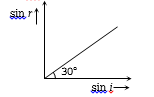(a)   1.5

(b)   2

(c)   2–1

(d)   3–1/2

Concept Questions :-

Refraction at plane surface
High Yielding Test Series + Question Bank - NEET 2020

Difficulty Level:

For a concave mirror, if the virtual image is formed, the graph between m and u is of the form :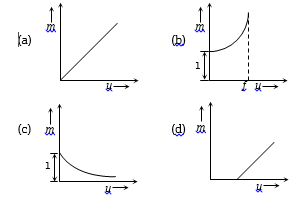Concept Questions :-

Reflection at spherical surface
High Yielding Test Series + Question Bank - NEET 2020

Difficulty Level:

For a convex lens, the distance of the object is taken on X-axis and the distance of the image is taken on Y-axis, the nature of the graph so obtained is :

(a) Straight line                      (b) Circle

(c) Parabola                           (d) Hyperbola

Concept Questions :-

Lenses